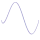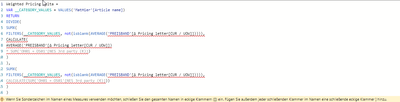cancel
Showing results for
Did you mean:Frequent Visitor

## Weighted average without blanks

Hello everybody,

I'm calculating a weighted average of a minimum price weighted by Sales on an article level.

There are however blanks in the list of minimum prices so I dont want to considers those articles at all in the calculation! As there is already "Keepfilters" used in the calculation, I cant apply another filter to exclude the blanks, any idea what to do?

Weighted Pricing Delta =
VAR __CATEGORY_VALUES = VALUES('MatHier'[Article name])
RETURN
DIVIDE(
SUMX(
KEEPFILTERS(__CATEGORY_VALUES),
CALCULATE(
AVERAGE('PREISBAND'[Δ Pricing letter[CUR / UOW]]])
* SUM('OH01 + OS01'[NES 3rd party (€)])
)
),
SUMX(
KEEPFILTERS(__CATEGORY_VALUES),
CALCULATE(SUM('OH01 + OS01'[NES 3rd party (€)]))
)
)
1 ACCEPTED SOLUTIONCommunity Support

You can use calculatetable() to adjust the VAR __CATEGORY_VALUES

VAR __CATEGORY_VALUES= CALCULATETABLE(VALUES('MatHier'[Article name]), ALLNOBLANKROW('MatHier'[minimum prices]))

If does not work, please try this code or share your pbix file without sensitive data.

or try this code:

``````Weighted Pricing Delta =
VAR _s =
SUMMARIZE (
'MatHier',
[Article name],
"avg", AVERAGE ( 'PREISBAND'[Δ Pricing letter[CUR / UOW]]] ),
"sum", SUM ( 'OH01 + OS01'[NES 3rd party (€)] )
)
VAR _1 =
SUMX ( FILTER ( _s, [avg] <> BLANK () ), [avg] * [sum] )
VAR _2 =
SUMX ( FILTER ( _s, [avg] <> BLANK () ), [sum] )
RETURN
DIVIDE ( _1, _2 )
``````

Best Regards

Community Support Team _ chenwu zhu

If this post helps, then please consider Accept it as the solution to help the other members find it more quickly.

3 REPLIES 3Community Support

You can use calculatetable() to adjust the VAR __CATEGORY_VALUES

VAR __CATEGORY_VALUES= CALCULATETABLE(VALUES('MatHier'[Article name]), ALLNOBLANKROW('MatHier'[minimum prices]))

If does not work, please try this code or share your pbix file without sensitive data.

or try this code:

``````Weighted Pricing Delta =
VAR _s =
SUMMARIZE (
'MatHier',
[Article name],
"avg", AVERAGE ( 'PREISBAND'[Δ Pricing letter[CUR / UOW]]] ),
"sum", SUM ( 'OH01 + OS01'[NES 3rd party (€)] )
)
VAR _1 =
SUMX ( FILTER ( _s, [avg] <> BLANK () ), [avg] * [sum] )
VAR _2 =
SUMX ( FILTER ( _s, [avg] <> BLANK () ), [sum] )
RETURN
DIVIDE ( _1, _2 )
``````

Best Regards

Community Support Team _ chenwu zhu

If this post helps, then please consider Accept it as the solution to help the other members find it more quickly.Super User

@lennardheyder , Try like

Weighted Pricing Delta =
VAR __CATEGORY_VALUES = VALUES('MatHier'[Article name])
RETURN
DIVIDE(
SUMX(
FILTERS(__CATEGORY_VALUES, not(isblank(AVERAGE('PREISBAND'[Δ Pricing letter[CUR / UOW]])))),
CALCULATE(
AVERAGE('PREISBAND'[Δ Pricing letter[CUR / UOW]])
* SUM('OH01 + OS01'[NES 3rd party (€)])
)
),
SUMX(
FILTERS(__CATEGORY_VALUES, not(isblank(AVERAGE('PREISBAND'[Δ Pricing letter[CUR / UOW]])))),
CALCULATE(SUM('OH01 + OS01'[NES 3rd party (€)]))
)
)Frequent VisitorFormula does not work unfortunatley....

Seems that "Filters" does not work with the input of a variableAnnouncements#### Exclusive opportunity for Women!

Join us for a free, hands-on Microsoft workshop led by women trainers for women where you will learn how to build a Dashboard in a Day!#### Power Platform Conference-Power BI and Fabric Sessions

Join us Oct 1 - 6 in Las Vegas for the Microsoft Power Platform Conference.Top Solution Authors
Top Kudoed Authors
Users online (3,049)